# 8 Results

View
Selected filters:
• Fractions Decimals and Percents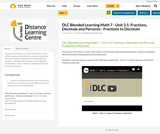Conditional Remix & Share Permitted
CC BY-NC-SA
Rating

The purpose of this lesson is to learn that numbers can be written in decimal and fraction form, as well as learning the difference between repeating and terminating decimals.

Included is a YouTube video to support Grade 7 Blended Learning Math - Unit 3.1: Fractions, Decimals and Percents - Fractions to Decimals.

Subject:
Math
Material Type:
Activity/Lab
Homework/Assignment
Lesson
Provider:
Sun West Distance Learning Centre (DLC)
Author:
Sun West School Division
06/07/2019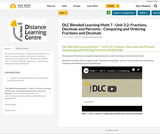Conditional Remix & Share Permitted
CC BY-NC-SA
Rating

The purpose of this lesson is to explore using benchmarks to order and compare fractions and decimals.

Included is a YouTube video to support Grade 7 Blended Learning Math - Unit 3.2: Fractions, Decimals and Percents - Comparing and Ordering Fractions and Decimals.

Subject:
Math
Material Type:
Activity/Lab
Homework/Assignment
Lesson
Provider:
Sun West Distance Learning Centre (DLC)
Author:
Sun West School Division
06/07/2019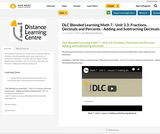Conditional Remix & Share Permitted
CC BY-NC-SA
Rating

The purpose of this lesson is to learn how to add and subtract decimals to the thousandths place value using from end estimation.

Included is a YouTube video to support Grade 7 Blended Learning Math - Unit 3.3: Fractions, Decimals and Percents - Adding and Subtracting Decimals.

Subject:
Math
Material Type:
Activity/Lab
Homework/Assignment
Lesson
Provider:
Sun West Distance Learning Centre (DLC)
Author:
Sun West School Division
06/07/2019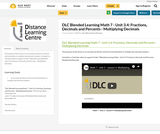Conditional Remix & Share Permitted
CC BY-NC-SA
Rating

The purpose of this lesson is to use base ten blocks and front end estimation to multiply two decimal numbers.

Included is a YouTube video to support Grade 7 Blended Learning Math - Unit 3.4: Fractions, Decimals and Percents - Multiplying Decimals.

Subject:
Math
Material Type:
Activity/Lab
Homework/Assignment
Lesson
Provider:
Sun West Distance Learning Centre (DLC)
Author:
Sun West School Division
06/07/2019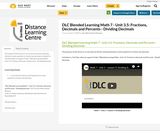Conditional Remix & Share Permitted
CC BY-NC-SA
Rating

The purpose of this lesson is to use base ten blocks and long division to solve question to involve two decimals.

Included is a YouTube video to support Grade 7 Blended Learning Math - Unit 3.5: Fractions, Decimals and Percents - Dividing Decimals.

Subject:
Math
Material Type:
Activity/Lab
Homework/Assignment
Lesson
Provider:
Sun West Distance Learning Centre (DLC)
Author:
Sun West School Division
06/07/2019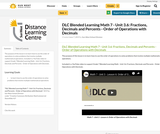Conditional Remix & Share Permitted
CC BY-NC-SA
Rating

The purpose of this lesson is to learn how to use the order of operations to solve problems that involve multiple mathematical operations.

Included is a YouTube video to support Grade 7 Blended Learning Math - Unit 3.6: Fractions, Decimals and Percents - Order of Operations with Decimals.

Subject:
Math
Material Type:
Activity/Lab
Homework/Assignment
Lesson
Provider:
Sun West Distance Learning Centre (DLC)
Author:
Sun West School Division
06/07/2019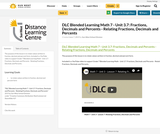Conditional Remix & Share Permitted
CC BY-NC-SA
Rating

The purpose of this lesson is to relate values written in fraction, decimal and percent form.

Included is a YouTube video to support Grade 7 Blended Learning Math - Unit 3.7: Fractions, Decimals and Percents - Relating Fractions, Decimals and Percents.

Subject:
Machining
Material Type:
Activity/Lab
Homework/Assignment
Lesson
Provider:
Sun West Distance Learning Centre (DLC)
Author:
Sun West School Division
06/07/2019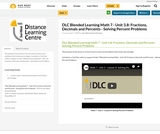Conditional Remix & Share Permitted
CC BY-NC-SA
Rating

The purpose of this lesson is to learn how to solve word problems that involve percentages.

Included is a YouTube video to support Grade 7 Blended Learning Math - Unit 3.8: Fractions, Decimals and Percents - Solving Percent Problems.

Subject:
Math
Material Type:
Activity/Lab
Homework/Assignment
Lesson
Provider:
Sun West Distance Learning Centre (DLC)
Author:
Sun West School Division